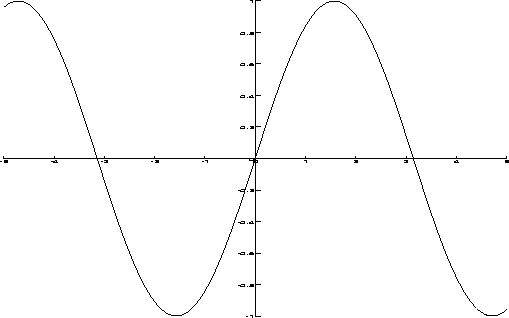Next: Superimposing Plots Up: Creating Plots Previous: Plotting a Set of

Plotting a Function and Range

DrawPlot(fnc : procedure, r : range)
The range r labels the x-axis and the function determines the values for the y-axis. We show a simple example which plots the sin function for the range -5..5.

> my_sine := proc(x)
>   return(sin(x));
> end;
> DrawPlot( my_sine, -5..5);

In the above example, we defined a function my_sine which tells Darwin how to treat each point in the range -5..5. Darwin allows us the following short hand notation.

> DrawPlot(x -> sin(x), -5..5);
The plot is shown in Figure.Gaston Gonnet
1998-09-15